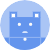search
Search
Publish
Guest 0reps
Thanks for the thanks!
close
account_circle
Profile
exit_to_app
Sign out
search
keyboard_voice
close
Searching Tips
Search for a recipe: "Creating a table in MySQL"
Search for an API documentation: "@append"
Search for code: "!dataframe"
Apply a tag filter: "#python"
Useful Shortcuts
/ to open search panel
Esc to close search panel
to navigate between search results
d to clear all current filters
Enter to expand content previewDoc SearchCode Search BetaSORRY NOTHING FOUND!
mic
Start speaking...Voice search is only supported in Safari and Chrome.
Shrink
Navigate to
A
A
share
thumb_up_alt
bookmark
arrow_backShareTwitterFacebook

# NumPy | std method

Programming
chevron_right
Python
chevron_right
NumPy
chevron_right
Documentation
schedule Mar 9, 2022
Last updated
local_offer PythonNumPy
Tags

NumPy's std(~) method computes the standard deviation of the values in the input array. The standard deviation is computed using the following formula:

$$\sqrt{\frac{1}{N}\sum_{i=0}^{N}\left(x_i-\bar{x}^2\right)}$$

Where,

• $N$ is the size of the given array (i.e. the sample size)

• $x_i$ is the value of the $i$th index in the NumPy array

• $\bar{x}$ is the sample mean

NOTE

The std(~) method can also compute the unbiased estimate of the standard deviation. We do this by setting ddof=1 in the parameters, as we shall see later in the examples.

# Parameters

1. a | array-like

The input array.

2. axislink | int or tuple | optional

The axis along which to compute the standard deviation. For 2D arrays, the allowed values are as follows:

Axis

Meaning

0

Standard deviation will be computed column-wise

1

Standard deviation will be computed row-wise

None

Standard deviation will be computed on a flattened array

By default, axis=None.

3. dtype | string or type | optional

The type used to compute the standard deviation. If the input array is of type int, then float32 will be used. If the input array is of another numerical type, then its type will be used.

4. ddoflink | int | optional

The delta degree of freedom. This can be used to modify the denominator in the front:

$$\sqrt{\frac{1}{N-ddof}\sum_{i=0}^{N}\left(x_i-\bar{x}^2\right)}$$

By default, ddof=0.

# Return value

An int representing the standard deviation of the provided values.

# Examples

## Standard deviation of a 1D array

 np.std([1,2,3,4]) 1.118 

## Computing sample standard deviation

To compute the sample standard deviation, set ddof=1:

 np.std([1,2,3,4], ddof=1) 1.290 

## Computing population standard deviation

To compute the population standard deviation, leave out the ddof parameter or explicitly set ddof=0:

 np.std([1,2,3,4]) # By default, ddof=0 1.118 

## Standard deviation of a 2D array

### Entire array

Without specifying the axis parameter, NumPy will just regard your NumPy array as a flattened array.

 np.std([[1,2],[3,4]]) 1.118 

This code is fundamentally the same as np.std([1,2,3,4]).

### Column-wise

To compute the standard deviation column-wise, specify axis=0 in the parameters:

 np.std([[1,4],[2,6], [3,8]], axis=0) array([0.81649658, 1.63299316]) 

Here, we're computing the standard deviation of [1,2,3] (i.e. the first column) as well as [4,6,8] (i.e. the second column).

### Row-wise

To compute the standard deviation column-wise, specify axis=1 in the parameters:

 np.std([[1,4],[2,6], [3,8]], axis=1) array([1.5, 2. , 2.5]) 

Here, we're computing three standard deviation: first row (i.e. [1,4]), second row (i.e. [2,6]) and third row (i.e. [3,8]).

WARNING

Sometimes the numerical type float32 may not be accurate enough for your needs. If your application requires more accurate numbers, then set dtype=np.float64 in the argument. This will take up more memory, but will provide a more accurate result.